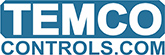# Simple In and Out - TB Module

Simple test to turn an output if input is on; and, set analog OUT9 to 10V (for a buzzer). However, no matter what input is turned on, OUT9 will only stay at 10V for 8-seconds and the it automatically turns off even though IN8 must be ON before it is turned off. Any ideas?

10 IF+ IN1 THEN OUT9 = 10
15 IF IN1 THEN START OUT1 ELSE STOP OUT1
20 IF+ IN2 THEN OUT9 = 10
25 IF IN2 THEN START OUT2 ELSE STOP OUT2
30 IF+ IN3 THEN OUT9 = 10
35 IF IN3 THEN START OUT3 ELSE STOP OUT3
40 IF+ IN4 THEN OUT9 = 10
45 IF IN4 THEN START OUT4 ELSE STOP OUT4
50 IF+ IN5 THEN OUT9 = 10
55 IF IN5 THEN START OUT5 ELSE STOP OUT5
60 IF+ IN6 THEN OUT9 = 10
65 IF IN6 THEN START OUT6 ELSE STOP OUT6
70 IF+ IN7 THEN OUT9 = 10
75 IF IN7 THEN START OUT7 ELSE STOP OUT7
80 IF IN8 = 1 THEN OUT9 = 0

Thanks,
JPotter

Got it. Used a VAR instead.

10 IF+ IN1 THEN VAR1 = 1
15 IF IN1 THEN START OUT1 ELSE STOP OUT1
20 IF+ IN2 THEN VAR1 = 1
25 IF IN2 THEN START OUT2 ELSE STOP OUT2
30 IF+ IN3 THEN VAR1 = 1
35 IF IN3 THEN START OUT3 ELSE STOP OUT3
40 IF+ IN4 THEN VAR1 = 1
45 IF IN4 THEN START OUT4 ELSE STOP OUT4
50 IF+ IN5 THEN VAR1 = 1
55 IF IN5 THEN START OUT5 ELSE STOP OUT5
60 IF+ IN6 THEN VAR1 = 1
65 IF IN6 THEN START OUT6 ELSE STOP OUT6
70 IF+ IN7 THEN VAR1 = 1
75 IF IN7 THEN START OUT7 ELSE STOP OUT7
80 IF IN8 THEN VAR1 = 0
90 IF VAR1 = 1 THEN OUT9 = 10 ELSE OUT9 = 0

Might be more informative to give these items names and set up an 'ALARMODE variable so that the logic flows more like a written sequence of operations.

10 REM **** WHEN AN INPUT IS ON, THE CORRESPONDING OUTPUT GOES ON
20 REM **** AND VICE VERSA.
30 OUT1 = IN1
40 OUT2 = IN2
50 OUT3 = IN3
60 OUT4 = IN4
70 OUT5 = IN5
80 OUT6 = IN6
90 OUT7 = IN7
100 REM ***** WHEN ANY OUTPUT GOES ON THE START THE ALARMODE VARIABLE
110 REM TURN OFF ALARMODE WHEN IN8 IS ON
130 IF+ IN1 THEN START ALARMODE
140 IF+ IN2 THEN START ALARMODE
150 IF+ IN3 THEN START ALARMODE
160 IF+ IN4 THEN START ALARMODE
170 IF+ IN5 THEN START ALARMODE
180 IF+ IN6 THEN START ALARMODE
190 IF+ IN7 THEN START ALARMODE
200 IF+ IN8 THEN STOP ALARMODE
210 REM ***** TURN ON THE BUZZER *****
220 IF ALARMODE THEN OUT9 = 10 ELSE OUT9 = 0

If this program doesn’t work then check around for other programs that might be affecting your outputs. Turn off all the other programs except for this one.

1 Like